Question

# 5.20 Figure 5.45 shows a motor mounted on a bracket that is hinged about the point...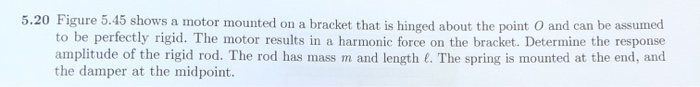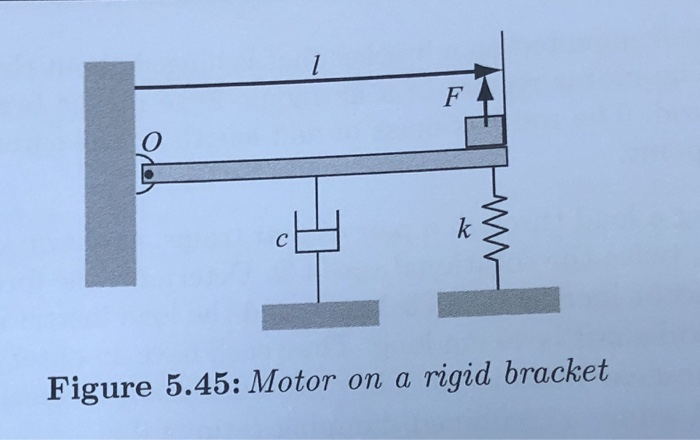5.20 Figure 5.45 shows a motor mounted on a bracket that is hinged about the point and can be assumed to be perfectly rigid. The motor results in a harmonic force on the bracket. Determine the response amplitude of the rigid rod. The rod has mass m and length. The spring is mounted at the end, and the damper at the midpoint.
! Figure 5.45: Motor on a rigid bracket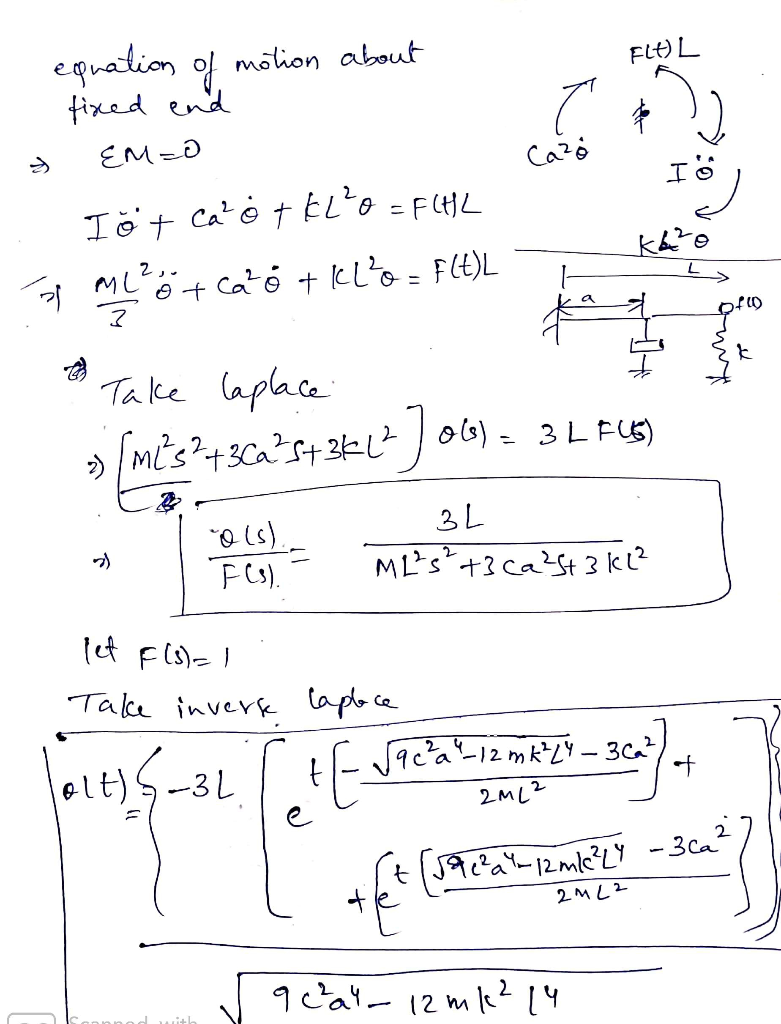#### Earn Coins

Coins can be redeemed for fabulous gifts.

Similar Homework Help Questions
• ### The figure (Figure 1) shows a model of a crane that may be mounted on a...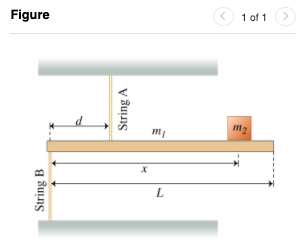The figure (Figure 1) shows a model of a crane that may be mounted on a truck.A rigid uniform horizontal bar of mass m1 = 90.0 kg and length L = 5.20 m is supported by two vertical massless strings. String A is attached at a distance d = 1.90 m from the left end of the bar and is connected to the top plate. String B is attached to the left end of the bar and is connected to...

• ### The figure (Figure 1) shows a model of a crane that may be mounted on a...

The figure (Figure 1) shows a model of a crane that may be mounted on a truck.A rigid uniform horizontal bar of mass = 100.0 and length = 5.800 is supported by two vertical massless strings. String A is attached at a distance = 1.600 from the left end of the bar and is connected to the top plate. String B is attached to the left end of the bar and is connected to the floor. An object of mass...

• ### The figure Figure 1 shows a model of a crane that may be mounted on a...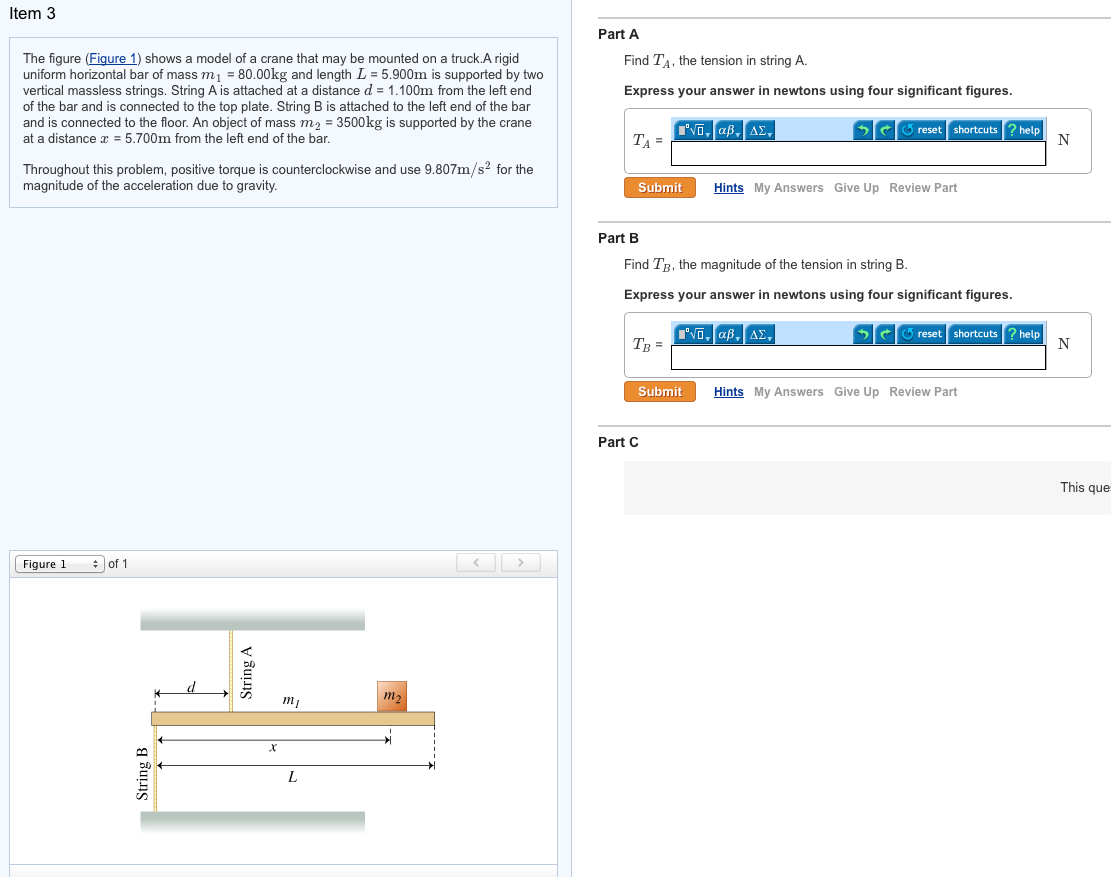The figure Figure 1 shows a model of a crane that may be mounted on a truck. A rigid uniform horizontal bar of mass m1 = 80.00kg and Length L = 5.900m is supported by two vertical massless strings. String A is attached at a distance d = 1.100m from the left end of the bar and is connected to the top plate. String B is attached to the left end of the bar and is connected to the floor....

• ### A small motor driven paint compressor set of mass 27 kg sits on rigid, massless platform...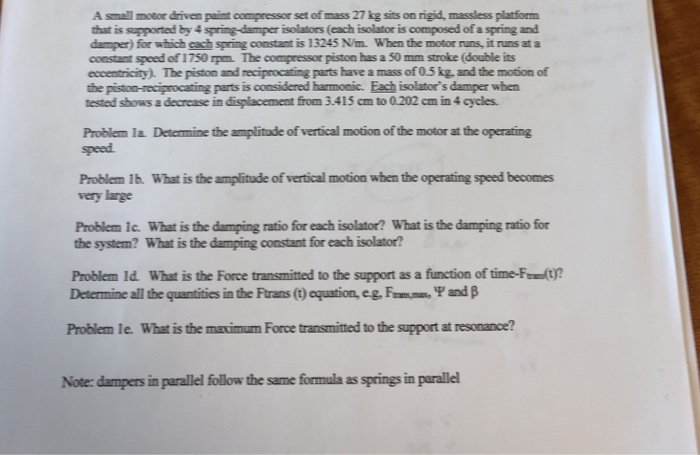A small motor driven paint compressor set of mass 27 kg sits on rigid, massless platform that is supported by 4 spring-damper isolators (each isolator is composed of a spring and damper) for which cach spring constant is 13245 N/m. When the motor runs, it runs at a constant speed of 1750 rpm. The compressor piston has a 50 mm stroke (double its eccentricity). The piston and reciprocating parts have a mass of 0.5 kg, and the motion of the...

• ### A slender, uniform metal rod of mass M and length l is pivoted without friction about an axis through its midpoint and p...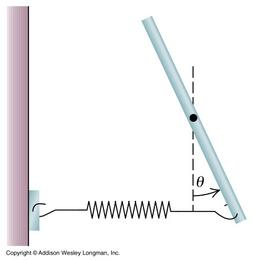A slender, uniform metal rod of mass M and length l is pivoted without friction about an axis through its midpoint and perpendicular to the rod. A horizontal spring, assumed massless and with force constant k, is attached to the lower end of the rod, with the other end of the spring attached to a rigid support. (Figure 1) 2. Find the torque τ due to the spring. Assume that θ is small enough that the spring remains effectively horizontal...

• ### The figure (Figure 1) shows a model of a crane that may be mounted on a...

The figure (Figure 1) shows a model of a crane that may be mounted on a truck.A rigid uniform horizontal bar of mass = 75.00 and length = 5.000 is supported by two vertical massless strings. String A is attached at a distance = 2.000 from the left end of the bar and is connected to the top plate. String B is attached to the left end of the bar and is connected to the floor. An object of mass...

• ### The figure shows a model of a crane that may be mounted on a truck. A...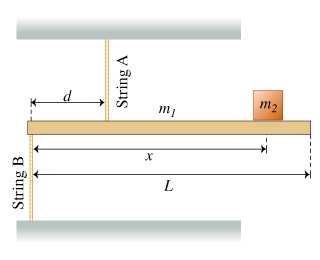The figure shows a model of a crane that may be mounted on a truck. A rigid uniform horizontal bar of mass shows a model of a crane that may be mounted on a truck. A rigid uniform horizontal bar of mass m_1 = 95.00 kg and length L = 6.000 m is supported by two vertical massless strings. String A is attached at a distance d = 1.300 m from the left end of the bar and is connected...

• ### A Pivoting Rod on a SpringA slender, uniform metal rod of mass and length is pivoted without friction about an axis through its midpoint andperpendicular to therod. A horizontal spring, assumed massless andwith force constant ,is attached to the lower end of the rod, with the other end of thespring attached to a rigid support.Assume that the spring is relaxed(exerts no torque on the rod) when the rod is vertical. The rod isdisplaced by a small angle from the vertical.Find the torque due to the...

• ### Vibration Engineering Figure Q5(b) shows a motor having mass of 50 kg is mounted on the...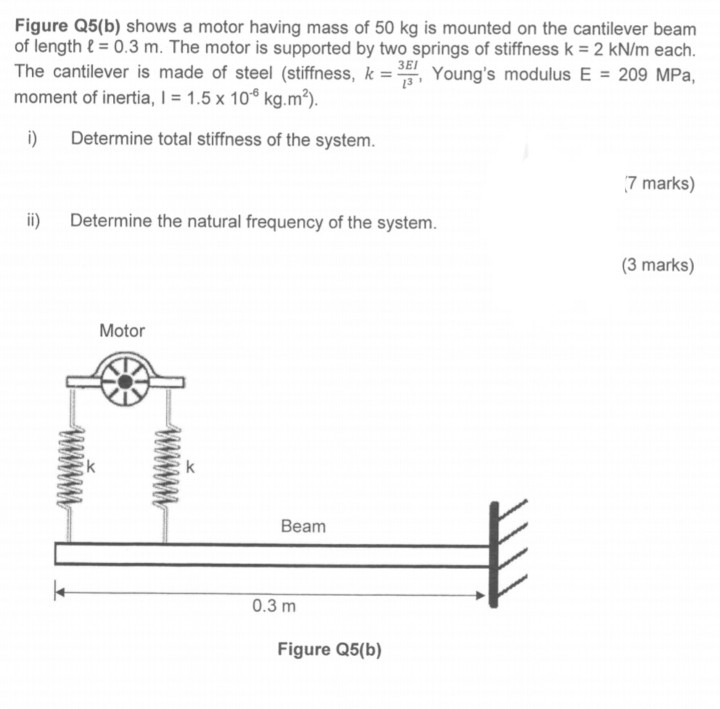Vibration Engineering Figure Q5(b) shows a motor having mass of 50 kg is mounted on the cantilever beam of length l = 0.3 m. The motor is supported by two springs of stiffness k = 2 kN/m each. The cantilever is made of steel (stiffness, k = 3E1 Young's modulus E = 209 MPa, moment of inertia, I = 1.5 x 10 kg.m²). i) Determine total stiffness of the system. 7 marks) ii) Determine the natural frequency of the system....

• ### The figure (Figure 1) shows a model of a crane that may be mounted on a...

The figure (Figure 1) shows a model of a crane that may be mounted on a truck.A rigid uniform horizontal bar of mass m1 = 85.0 kg and length L = 5.80 m is supported by two vertical massless strings. String A is attached at a distance d = 2.00 m from the left end of the bar and is connected to the top plate. String B is attached to the left end of the bar and is connected to...